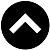Type something and hit enter

# 净利与净利率

(Profit Margin = Net Profit/Revenue x 100%)
Harta 2018年净利率 = 439.4m/2405.63m x 100% = 18.26%
TopGlov 2018年净利率 = 433.6m/4213.99m x 100% = 10.29%
Kossan 2017年净利率  =  185.58m/1957.44m x 100% = 9.48%
Supermx 2018年净利率 = 107.21m/1304.46m x 100% = 8.22%

PIE 2017 年净利率 = 48.01m/679.28m x 100% = 7.07% , PE = 14.91
FPI 2017 年净利率 = 42.31m/462.64m x 100% = 9.14%, PE = 9.21
VS 2018 年净利率 = 150.77m/4089.19m x 100% = 3.69%, PE = 18.93
SKPRes 2018 年净利率 = 127.1m/2104.5m x 100% = 6.04%, PE = 12.98

*注:从2012到2017，Gagr的年数是5而不是6。有不少朋友都有察觉我在营收一文中的错误并指正，让我感到欣慰。我一直认为，研究股票需要小心查证而不该照单全收。尤其是在读投行的研究报告时，在投资前小心查证并自己多做一次功课，对学习基本面功夫必有助益。

https://tengkhan.blogspot.com/2018/10/blog-post_13.html